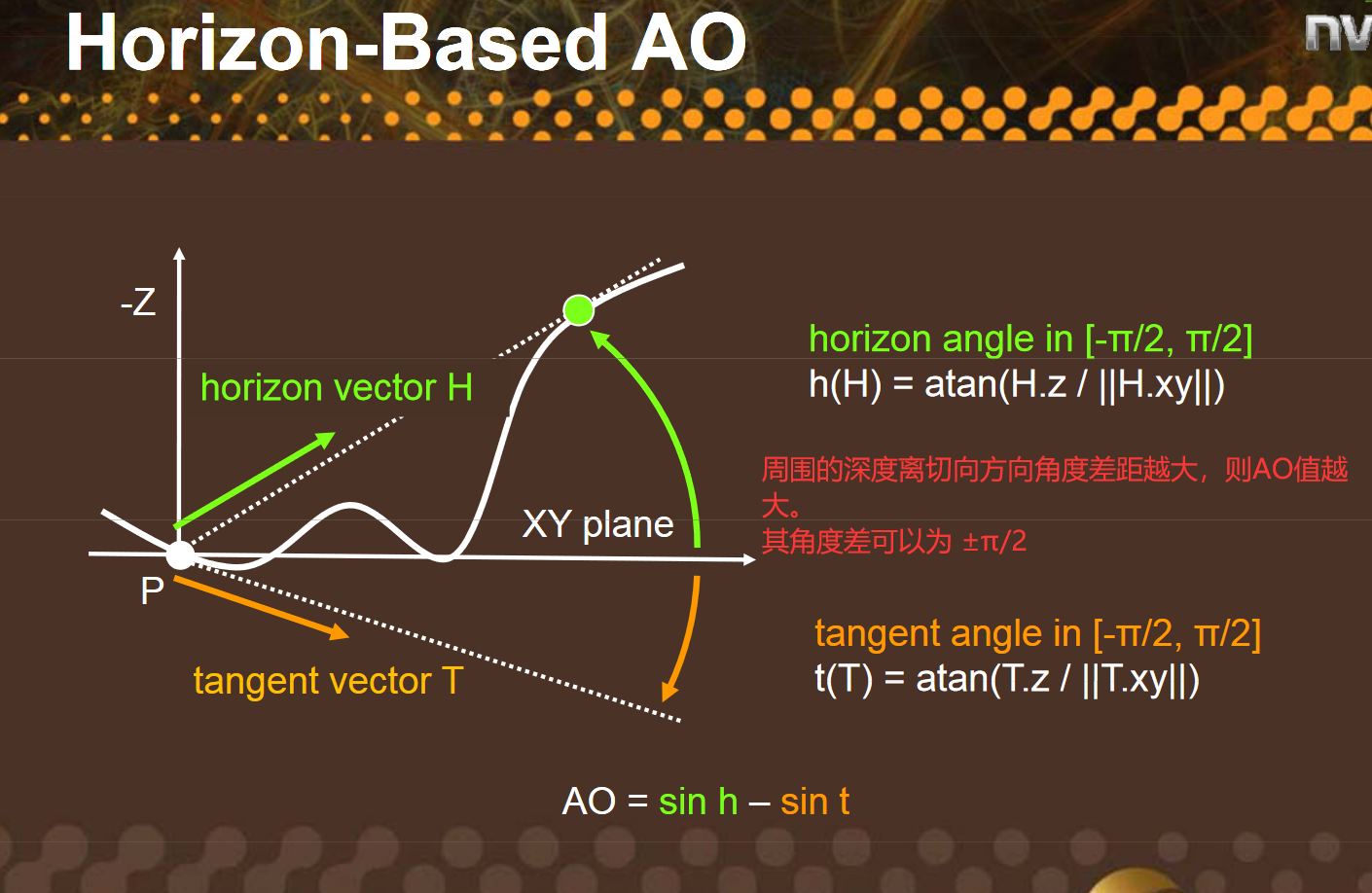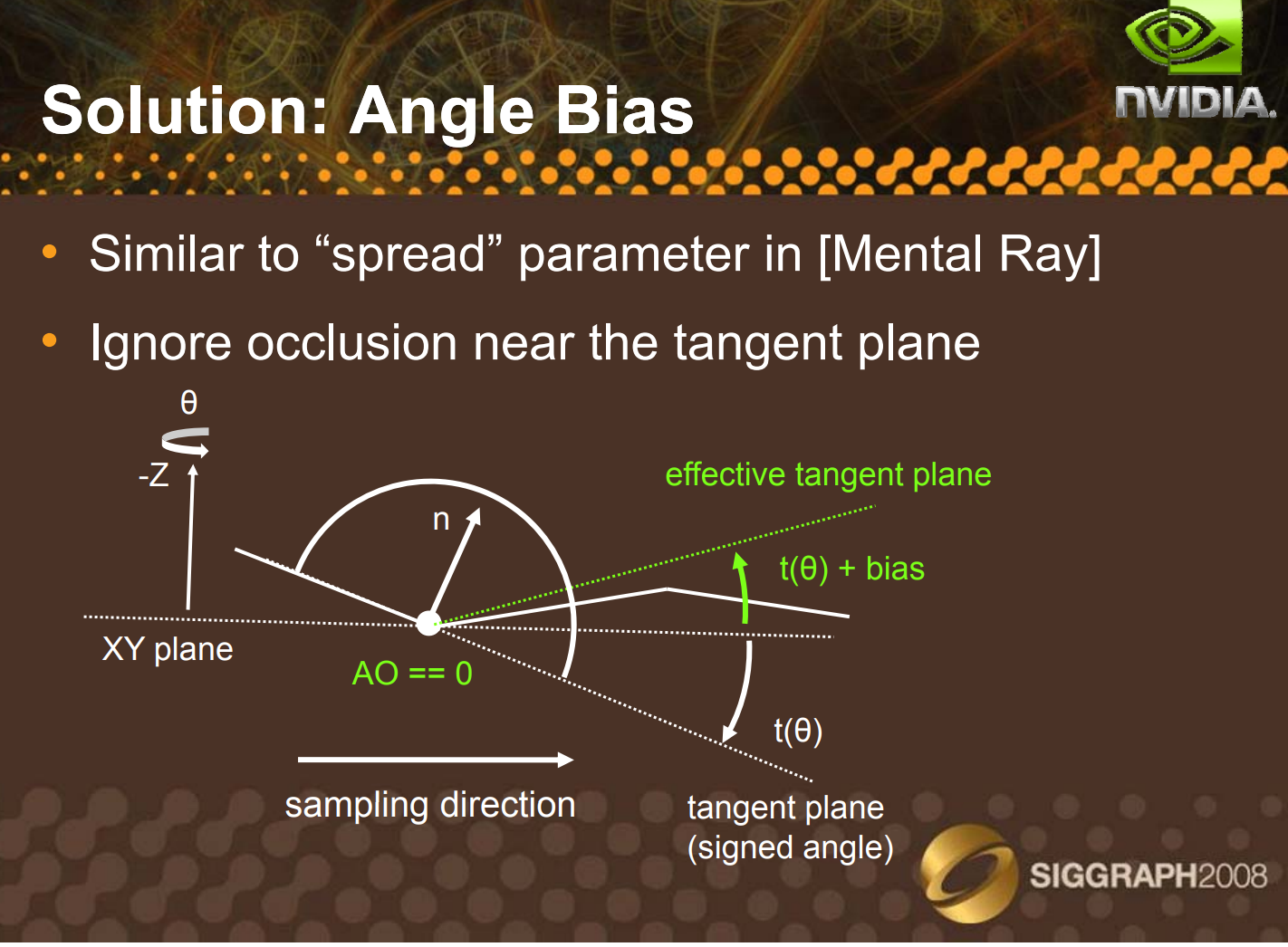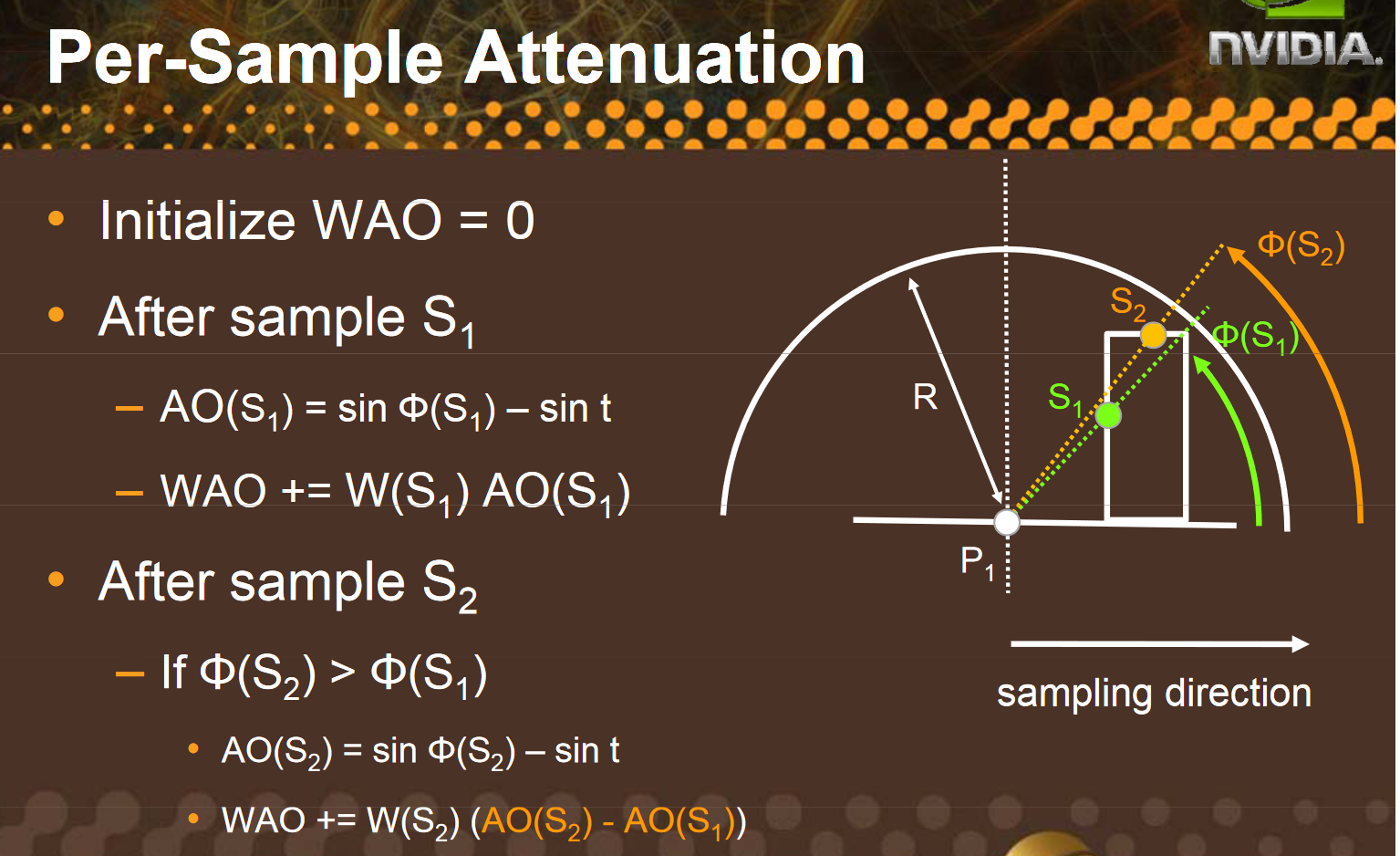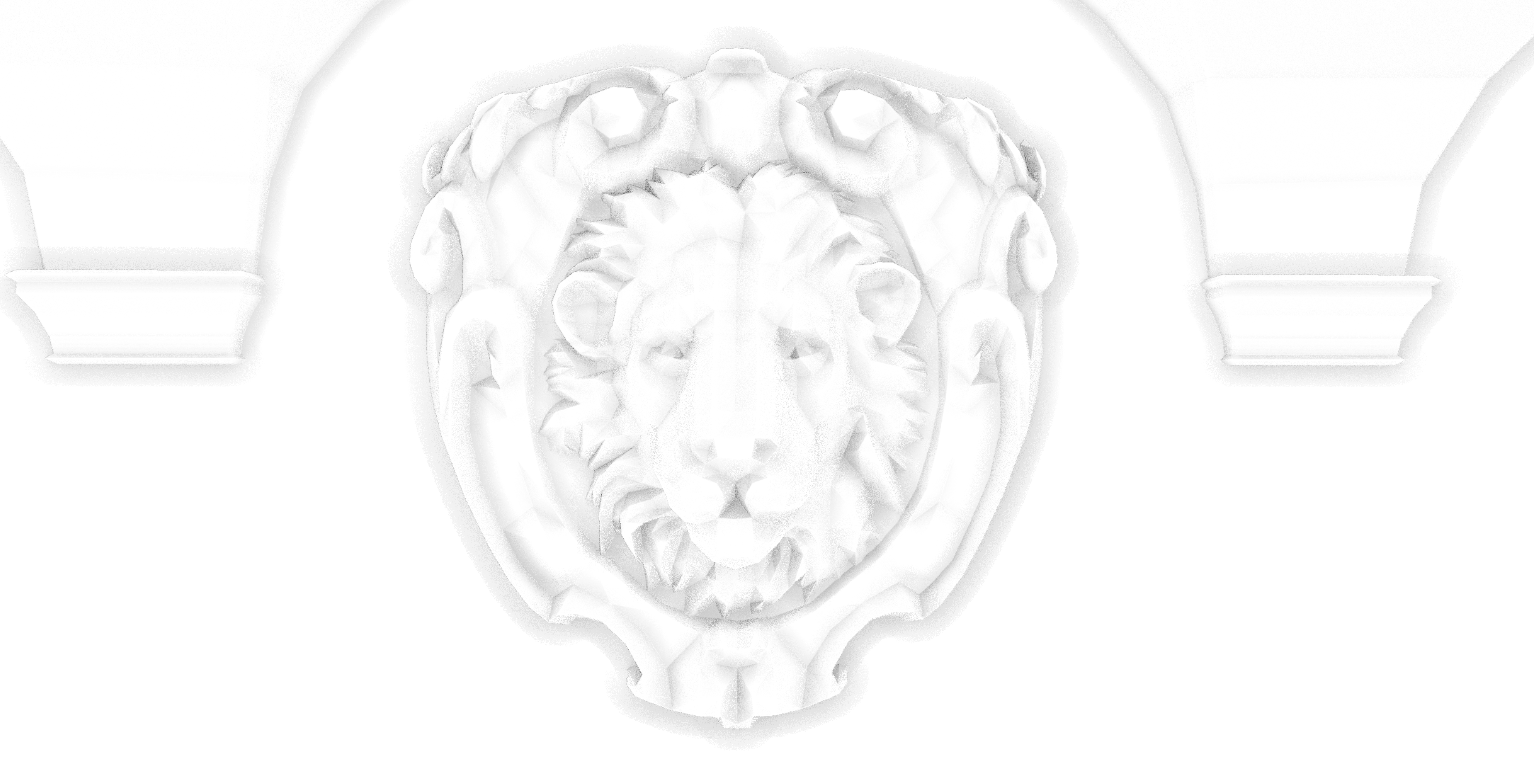HBAO的Unity实现杂记 | Blurred code

# HBAO的Unity实现杂记

2022/10/15

LastMod:2022/10/16

Categories: CG

## HBAO实现杂记### Tangent Bias``````float3 tangent =
FetchViewPos(input.uv + dir * _MainTex_TexelSize.xy) -
FetchViewPos(input.uv - dir * _MainTex_TexelSize.xy);
tangent = normalize(tangent);
``````

### 距离加权采样`AO(S2) - AO(S1) = sin(S2) - sint`

1. 需要有一个Top变量追踪当前最大的`sin(theta) - sin(Tanget)`，初始化为0
2. 每个步进只计算比当前AO大的点
3. 越靠近半圆的边界，对AO的贡献越低
``````inline float fullAO(float3 pos, float3 stepPos, float3 normal,float3 tangent ,inout float top)
{
float3 h = stepPos - pos;
float3 h_dir = normalize(h);
// 计算采样点的sin
float tanH = ViewPosTangent(h_dir);
float sinH = TanToSin(tanH);
// 计算Tanget的sin
float tanT = BiasedViewPosTanget(tangent,_AOBias);
float sinT = TanToSin(tanT);

// 当前采样的AO值
float sinBlock = sinH - sinT;
// 如果低于之前的采样点，就是0，如果比之前的采样点大，则计算它的贡献(但是要算上距离衰减)
float diff = max(sinBlock - top, 0);
top = max(sinBlock, top);

// 计算采样点距离采样中心的距离，衰减为 1 - d^2/r^2
float dist = length(h);
return diff * FallOff(dist);
}
``````

# 实现上的差异

1. PPT第46页提到的`Snap UV`在代码中似乎会引入画面出现某种奇怪的花纹，也许是我没实现正确。使用`Bilinear Sampling`没有看到肉眼可见的瑕疵，所以没有做。
2. PPT第27页提到的`DepthAware Blur`没有做，换成了简单的Gaussian Blur，会造成边缘有点模糊。